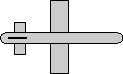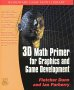# Maths - Conversion Euler To Matrix

## Definition of terms:

This depends on what conventions are used for the Euler Angles. The following are derived on the euler angle page, the first assumes NASA Standard Airplane:

[R] =
 c θ *c φ -c θ *s φ s θ cψ*sφ + s ψ* s θ*c φ cψ*cφ -s ψ*s θ*s φ -s ψ*c θ sψ*sφ - c ψ* s θ*c φ sψ*cφ + c ψ*s θ*s φ c ψ*c θ

Or, from the same page, this uses NASA Standard Airplane taking angles in the reverse order:

[R] =
 c ψ*c θ sψ*cφ + c ψ*s θ*s φ sψ*sφ - c ψ* s θ*c φ -s ψ*c θ cψ*cφ -s ψ*s θ*s φ cψ*sφ + s ψ* s θ*c φ s θ -c θ *s φ c θ *c φ

Java code to do conversion:

```/** this conversion uses NASA standard aeroplane conventions as described on page:
*   https://www.euclideanspace.com/maths/geometry/rotations/euler/index.htm
*   Coordinate System: right hand
*   Positive angle: right hand
*   matrix row column ordering:
*   [m00 m01 m02]
*   [m10 m11 m12]
*   [m20 m21 m22]*/
public final void rotate(double heading, double attitude, double bank) {    // Assuming the angles are in radians.    double c1 = Math.cos(heading);    double s1 = Math.sin(heading);    double c2 = Math.cos(attitude);    double s2 = Math.sin(attitude);    double c3 = Math.cos(bank);    double s3 = Math.sin(bank);
m00 = c1 * c2;    m01 = -s1 * c2;    m02 = s2;    m10 = s1 * c3+(c1 * s2 * s3);    m11 = (c1*c3) - (s1 * s2 * s3);    m12 = -c2 * s3;    m20 = (s1 * s3) - (c1 * s2 * c3);    m21 = (c1 * s3) + (s1 * s2 * c3);    m22 = c2*c3;}

```

## Example

 we take the 90 degree rotation from this:to this:As shown here the axis angle for this rotation is:

bank = 90 degrees
attitude = 0 degrees

so substituteing this in the above formula gives:

• c θ =cos(heading) = 1
• c φ =cos(attitude) = 1
• cψ =cos(bank) = 0
• s θ = sin(heading) = 0
• s φ =sin(attitude) = 0
• s ψ =sin(bank) = 1
[R] =
 c θ *c φ -c θ *s φ s θ cψ*sφ + s ψ* s θ*c φ cψ*cφ -s ψ*s θ*s φ -s ψ*c θ sψ*sφ - c ψ* s θ*c φ sψ*cφ + c ψ*s θ*s φ c ψ*c θ
[R] =
 1 0 0 0 0 -1 0 1 0

This agrees with the matix rotations here.

 metadata block see also: Correspondence about this page Book Shop - Further reading. Where I can, I have put links to Amazon for books that are relevant to the subject, click on the appropriate country flag to get more details of the book or to buy it from them.3D Math Primer - Aimed at complete beginners to vector and matrix algebra.

This site may have errors. Don't use for critical systems.